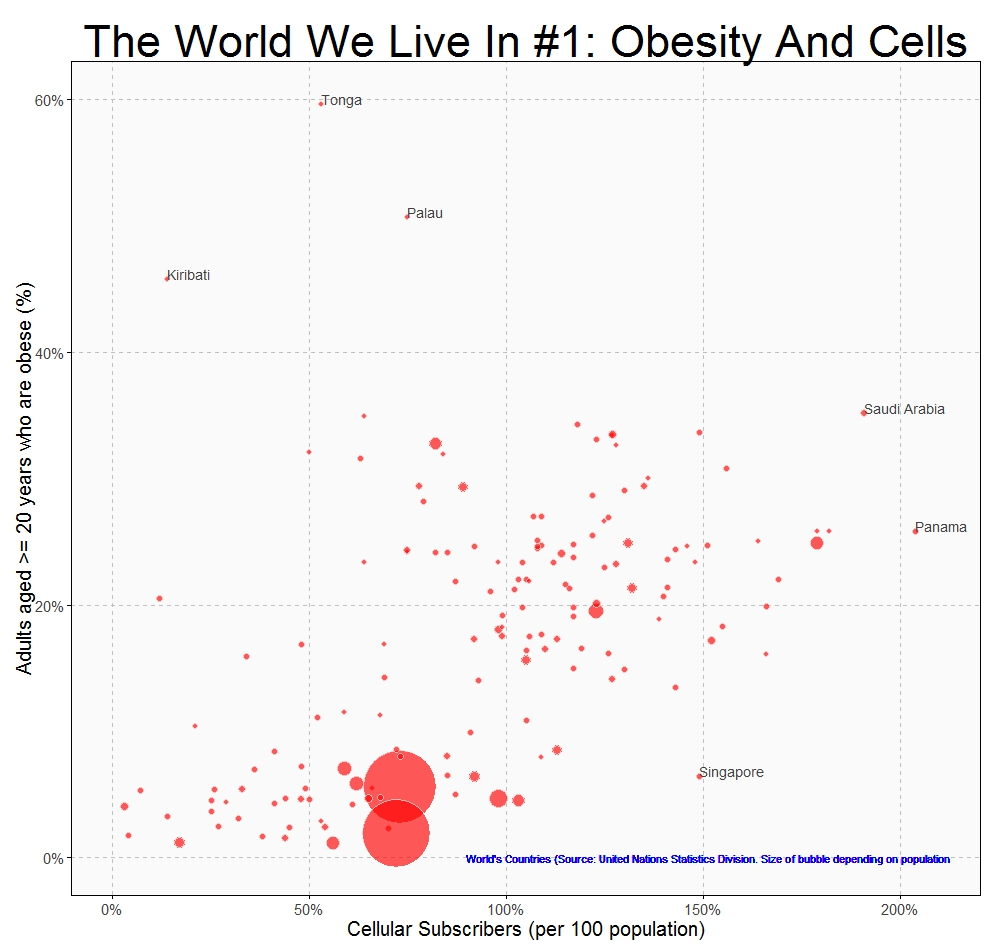# The World We Live In #5: Calories And Kilograms

I enjoy doing new tunes; it gives me a little bit to perk up, to pay a little bit more attention (Earl Scruggs, American musician)

I recently finished reading The Signal and the Noise, a book by Nate Silver, creator of the also famous FiveThirtyEight blog. The book is a very good reading for all data science professionals, and is a must in particular for all those who work trying to predict the future. The book praises the bayesian way of thinking as the best way to face and modify predictions and criticizes rigid ways of thinking with many examples of disastrous predictions. I enjoyed a lot the chapter dedicated to chess and how Deep Blue finally took over Kasparov. In a nutshell: I strongly recommend it.
One of the plots of Silver’s book present a case of false negative showing the relationship between obesity and calorie consumption across the world countries. The plot shows that there is no evidence of a connection between both variables. Since it seemed very strange to me, I decided to reproduce the plot by myself.

I compared these two variables:

• Dietary Energy Consumption (kcal/person/day) estimated by the FAO Food Balance Sheets.
• Prevalence of Obesity as percentage of defined population with a body mass index (BMI) of 30 kg/m2 or higher estimated by the World Health Organization

And this is the resulting plot:As you can see there is a strong correlation between two variables. Why the experiment of Nate Silver shows the opposite? Obviously we did not plot the same data (although, in principle, both of us went to the same source). Anyway: to be honest, I prefer my plot because shows what all of we know: the more calories you eat, the more weight you will see in your bathroom scale. Some final thoughts seeing the plot:

• I would like to be Japanese: they don’t gain weight!
• Why US people are fatter than Austrian?
• What happens in Samoa?

Here you have the code to do the plot:

```library(xlsx)
library(dplyr)
library(ggplot2)
library(scales)
calories = read.xlsx(file="FoodConsumptionNutrients_en.xls", startRow = 4, colIndex = c(2,6), colClasses = c("character", "numeric"), sheetName="Dietary Energy Cons. Countries", stringsAsFactors=FALSE)
colnames(calories)=c("Country", "Kcal")
url_population = "http://esa.un.org/unpd/wpp/DVD/Files/1_Excel%20(Standard)/EXCEL_FILES/1_Population/WPP2015_POP_F01_1_TOTAL_POPULATION_BOTH_SEXES.XLS"
population = read.xlsx(file="Population.xls", startRow = 17, colIndex = c(3,71), colClasses = c("character", "numeric"), sheetName="ESTIMATES", stringsAsFactors=FALSE)
colnames(population)=c("Country", "Population")
# http://apps.who.int/gho/data/node.main.A900A?lang=en
url_obesity = "http://apps.who.int/gho/athena/data/xmart.csv?target=GHO/NCD_BMI_30A&profile=crosstable&filter=AGEGROUP:*;COUNTRY:*;SEX:*&x-sideaxis=COUNTRY&x-topaxis=GHO;YEAR;AGEGROUP;SEX&x-collapse=true"
obesity %>% select(matches("Country|2014.*Both")) -> obesity
colnames(obesity)=c("Country", "Obesity")
obesity %>% filter(Obesity!="No data") -> obesity
obesity %>% mutate(Obesity=as.numeric(substr(Obesity, 1, regexpr(pattern = "[[]", obesity\$Obesity)-1))) -> obesity
population %>% inner_join(calories,by = "Country") %>% inner_join(obesity,by = "Country") -> data
opts=theme(
panel.background = element_rect(fill="gray98"),
panel.border = element_rect(colour="black", fill=NA),
axis.line = element_line(size = 0.5, colour = "black"),
axis.ticks = element_line(colour="black"),
panel.grid.major = element_line(colour="gray75", linetype = 2),
panel.grid.minor = element_blank(),
axis.text = element_text(colour="gray25", size=15),
axis.title = element_text(size=18, colour="gray10"),
legend.key = element_blank(),
legend.position = "none",
legend.background = element_blank(),
plot.title = element_text(size = 40, colour="gray10"))
ggplot(data, aes(x=Kcal, y=Obesity/100, size=log(Population), label=Country), guide=FALSE)+
geom_point(colour="white", fill="sandybrown", shape=21, alpha=.55)+
scale_size_continuous(range=c(2,40))+
scale_x_continuous(limits=c(1500,4100))+
scale_y_continuous(labels = percent)+
labs(title="The World We Live In #5: Calories And Kilograms",
x="Dietary Energy Consumption (kcal/person/day)",
y="% population with body mass index >= 30 kg/m2")+
geom_text(data=subset(data, Obesity>35|Kcal>3700), size=5.5, colour="gray25", hjust=0, vjust=0)+
geom_text(data=subset(data, Kcal<2000), size=5.5, colour="gray25", hjust=0, vjust=0)+
geom_text(data=subset(data, Obesity<10 & Kcal>2600), size=5.5, colour="gray25", hjust=0, vjust=0)+
geom_text(aes(3100, .01), colour="gray25", hjust=0, label="Source: United Nations (size of bubble depending on population)", size=4.5)+opts
```

# The World We Live In #1: Obesity And Cells

Lesson learned, and the wheels keep turning (The Killers – The world we live in)

I discovered this site with a huge amount of data waiting to be analyzed. The first thing I’ve done is this simple graph, where you can see relationship between cellular subscribers and obese people. Bubbles are countries and its size depends on the population:Some quick conclusions:

• The more cellular subscribers, the more obese people
• Pacific islands such as Kiribati, Palau and Tonga are plenty of happy people
• Singapore people are thinner than they should be
• How do Saudi Arabian and Panamanian manage two cellulars?

This is the world we live in.

```cellular  =read.csv("UNdata_Export_20140930_cellular.csv",   nrows=193,   header=T, row.names=NULL)
require("sqldf")
require("plyr")
population=rename(population, replace = c("Country.or.Area" = "Country"))
population=sqldf("SELECT a.Country, a.Year, a.Value as Population
FROM population a INNER JOIN (SELECT Country, MAX(Year) AS Year FROM population GROUP BY 1) b
ON (a.Country=b.Country AND a.Year=b.Year)")
cellular=rename(cellular, replace = c("Country.or.Area" = "Country"))
cellular=rename(cellular, replace = c("Value" = "Cellular"))
obese=rename(obese, replace = c("Country.or.Area" = "Country"))
obese=rename(obese, replace = c("Year.s." = "Year"))
obese=sqldf("SELECT a.Country, a.Year, SUBSTR(TRIM(Value), 1, CHARINDEX(' [', TRIM(Value))) as Obeses
FROM obese a INNER JOIN (SELECT Country, MAX(Year) AS Year FROM obese WHERE GENDER='Both sexes' GROUP BY 1) b
ON (a.Country=b.Country AND a.Year=b.Year AND a.GENDER='Both sexes')")
obese\$Obeses=as.numeric(obese\$Obeses)
data=sqldf("SELECT a.Country, a.Cellular, c.Obeses, b.Population FROM cellular a inner join population b on a.Country = b.Country
inner join obese c on (a.Country = c.Country) WHERE a.Country NOT IN ('World', 'South Asia')")
require(ggplot2)
require(scales)
opts=theme(
panel.background = element_rect(fill="gray98"),
panel.border = element_rect(colour="black", fill=NA),
axis.line = element_line(size = 0.5, colour = "black"),
axis.ticks = element_line(colour="black"),
panel.grid.major = element_line(colour="gray75", linetype = 2),
panel.grid.minor = element_blank(),
axis.text.y = element_text(colour="gray25", size=15),
axis.text.x = element_text(colour="gray25", size=15),
text = element_text(size=20),
legend.key = element_blank(),
legend.position = "none",
legend.background = element_blank(),
plot.title = element_text(size = 45)
)
ggplot(data, aes(x=Cellular/100, y=Obeses/100, size=Population, label=Country), guide=FALSE)+
geom_point(colour="white", fill="red", shape=21, alpha=.65)+
scale_size_continuous(range=c(3,35))+
scale_x_continuous(limits=c(0,2.1), labels = percent)+
scale_y_continuous(limits=c(0,.6), labels = percent)+
labs(title="The World We Live In #1: Obesity And Cells",
x="Cellular Subscribers (per 100 population)",
y="Adults aged >= 20 years who are obese (%)")+
geom_text(data=subset(data, Cellular/100 > 1.9 | Obeses/100 > .4 | (Cellular/100 > 1.4 & Obeses/100 < .15)), size=5, colour="gray25", hjust=0, vjust=0)+
geom_text(aes(.9, .0), colour="blue", hjust=0, label="World's Countries (Source: United Nations Statistics Division. Size of bubble depending on population", size=4)+
opts
```